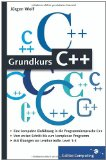# Swap two numbers in c ++

## Pointer (function void swap), C ++School> Computer Science> Programming Languages> C and C ++> Pointer (function void swap), C ++### POINTER

Write a Function void swap (int * p, int * q), which does the following:

When fde main function the declaration and definition of 2 int variables a and b contains:
int a = 10;

int b = 15;
and you in main also 2 int pointer Declare z1 and z2 so that z1 points to a and z2 to b, then after the call exchange(z1, z2) then point z1 to b and z2 to a without changing the values ​​of a and b! Before calling exchange(z1, z2) should contain the addresses of a, b, z1, z2 as well as the values ​​of the pointer z1 and z2 themselves and the contents of the memory cells pointed to by z1 and z2 are output. After this To deceive (for comparison) the addresses of z1 and z2 as well as z1 and z2 themselves and the content of the memory cells pointed to by z1 and z2 should be output. (Then the addresses of z1 and z2 would have to be unchanged, the values ​​of z1 and z2 themselves would have to be the addresses of a and b, but afterwards reversed!)
#include
int main ()
int a = 10;
int b = 15;
int * z1, * z2;
z1 = & a;
z2 =? // z1 and z2 declared as int, point to a and b
cout << "Address of a:" << & a << endl;
cout << "address of b:" << & b << endl;
cout << "value of a:" << a << endl;
cout << "value of b:" << b << endl;
cout << "Content of z1:" << * z1 << endl;
cout << "Content of z2:" << * z2 << endl;
int * temp;
temp = z1; // temp records the content of address z1
z1 = z2; // the content of z1 is saved as the content of z2
z2 = temp; // z2 is the content of temp
cout << "Address of a:" << & a << endl;
cout << "address of b:" << & b << endl;
cout << "value of a:" << a << endl;
cout << "value of b:" << b << endl;
cout << "Content of z1:" << * z1 << endl;
cout << "Content of z2:" << * z2 << endl;
return 0;

Write a function void exchange (int * p, int * q) that does the following:
If fde main function contains the declaration and definition of 2 int variables a and b:
int a = 10;
int b = 15;
and you also declare 2 int pointers z1 and z2 in main, so that z1 points to a and z2 to b, then after the call Swap (z1, z2) then z1 should point to b and z2 to a without them having to use the Have changed values ​​of a and b! Before calling Swap (z1, z2), the addresses of a, b, z1, z2 as well as the values ​​of the pointers z1 and z2 themselves and the content of the memory cells pointed to by z1 and z2 should be output. After swapping, the addresses of z1 and z2 as well as z1 and z2 themselves and the contents of the memory cells pointed to by z1 and z2 should be output (for comparison). (Then the addresses of z1 and z2 would have to be unchanged, the values ​​of z1 and z2 themselves would have to be the addresses of a and b, but swapped afterwards!) (354 words)
0 further documents on the subject of "C and C ++"
99 discussions on the topic in the forum
When using this document please cite it as: "Pointer (function void exchange), C ++", https://e-haushaben.de/Haushaben/D1628-Zeiger-CPlusPlus.php, accessed on 23.05.2021 at 11:14 pm

This is an external, user-generated content for which no liability is assumed.